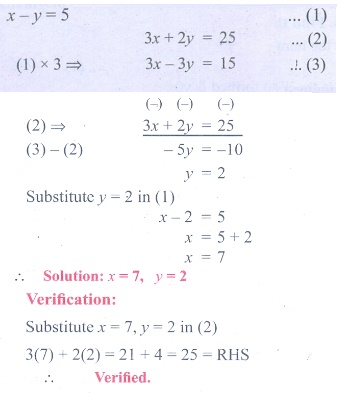Home | | Maths 9th std | Exercise 3.12: Solving by Elimination Method

# Exercise 3.12: Solving by Elimination Method

Maths : Algebra: Book Back, Exercise, Example Numerical Question with Answers, Solution : Exercise 3.12: Elimination Method of Solving simultaneous linear equations in Two Variables :

## Elimination Method of Solving simultaneous linear equations in Two Variables

Exercise 3.12

1. Solve by the method of elimination

(i) 2xy = 3; 3x + y = 7(ii) xy = 5; 3x + 2y = 25(iii) x/10 + y/5 = 14; x/8 + y/6 = 15(iv) 3(2x + y= 7xy; 3(x + 3y= 11xy(v) 4/x + 5y = 7; 3/x + 4 y = 5(vi) 13x + 11y = 70; 11x + 13y = 742. The monthly income of A and B are in the ratio 3:4 and their monthly expenditures are in the ratio 5:7. If each saves ₹ 5,000 per month, find the monthly income of each.3. Five years ago, a man was seven times as old as his son, while five year hence, the man will be four times as old as his son. Find their present age.Tags : Solving simultaneous linear equations in Two Variables | Numerical Problems with Answers, Solution | Algebra | Maths , 9th Maths : UNIT 3 : Algebra
Study Material, Lecturing Notes, Assignment, Reference, Wiki description explanation, brief detail
9th Maths : UNIT 3 : Algebra : Exercise 3.12: Solving by Elimination Method | Solving simultaneous linear equations in Two Variables | Numerical Problems with Answers, Solution | Algebra | Maths